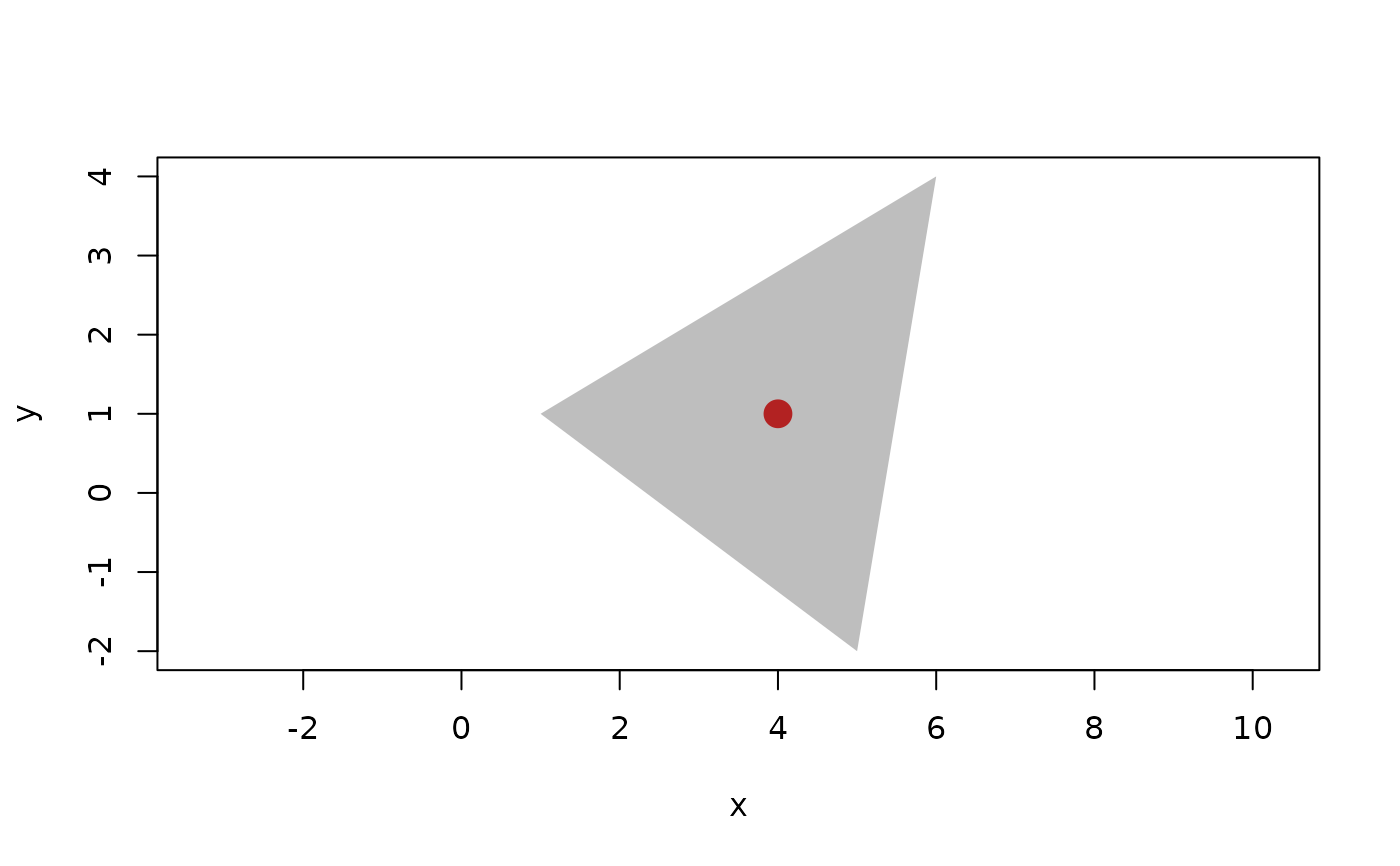The centroid is the geometric mean of the vertices in a geometry. euclid supports centroid calculations for triangles, tetrahedrons and 2-4 points

## Usage

centroid(x, ...)

## Arguments

x

A vector of triangles or tetrahedrons, or points if y, z, or t are provided

...

arguments passed on to methods. Specifically the method for euclid_geometry vectors accept up to three additional vectors of euclid_point (y, z, and t arguments) if x is a euclid_point vector

## Value

a vector of points

Other Locations: barycenter(), bisector(), circumcenter(), equidistant_line(), point(), radical(), weighted_point()

## Examples

p <- point(c(1, 5, 6), c(1, -2, 4))
# centroid of triangle
t <- triangle(p, p, p)
centroid(t)
#> <2D points >
#>  <x:4, y:1>

plot(t, col = "grey", border = NA)
euclid_plot(centroid(t), pch = 16, cex = 2, col = "firebrick")# Same as providing the points directly
centroid(p, p, p)
#> <2D points >
#>  <x:4, y:1>